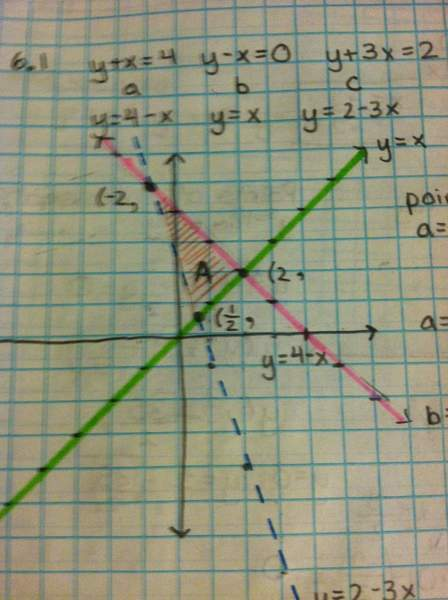# Find the area between three curves

MissEuropa

## Homework Statement

Sketch the region enclosed by the curves and compute its area as an integral along the x or y axis.
y+x=4 y-x=0 y+3x=2

## Homework Equations

top function - bottom function dx OR right function-left function dy

## The Attempt at a Solution

I originally had chosed to integrate with respect to y, I solved all equations for y as follows:
y=4-x y=x y=2-3x

I set all equations equal to each other to find three intersection x values: These are
x=2, x=-2, and x=1/2

This makes since considering the way I sketched the graph: see attachment.Now the Area is shaded in orange and labeled A: to set up the integral I did:
1/2
(4-x)-(2-3x) dx = 2x+x2 evaluated from -2 to 1/2
-2

My answer to this integral was (5/4)

Then I took this integral:
2
(4-x)-(x) = 4x-x2 evaluated from 1/2 to 2
1/2

My answer to this integral was (7/4)

It was olvious to me at this point that something went wrong because (5/4)-(7/4)=-(1/2) and areas cannot be negative.

This is the first time I've attempted to find the area between three curves. I am not sure how to arrange the integrals and which one is considered "top" or "bottom" because the graph that I drew shows the lower functions switching, which I tried to reflect in my calculations.

I'm really working hard to wrap my head around this stuff, so any help would be greatly appreciated.

Staff Emeritus
Homework Helper
Gold Member

## Homework Statement

Sketch the region enclosed by the curves and compute its area as an integral along the x or y axis.
y+x=4 y-x=0 y+3x=2

## Homework Equations

top function - bottom function dx OR right function-left function dy

## The Attempt at a Solution

I originally had chosed to integrate with respect to y, I solved all equations for y as follows:
y=4-x y=x y=2-3x

I set all equations equal to each other to find three intersection x values: These are
x=2, x=-2, and x=1/2
The lines y=4-x , and y=2-3x intersect at x = -1 not at x = -2.

By the way, the lines y=4-x, and y=x are perpendicular to each other, so it's easy to check the answer.

MissEuropa
Thanks, I wouldn't of caught that.
So I've edited my limits of integration:
1/2
((4-x)-(2-3x)) = 4.25
-1

2
((4-x)-(x))= (7/4)
1/2

4.25-(7/4) = 2.5

Alas, I am still incorrect. Is there something else I am missing?

Thanks again.

Staff Emeritus
Homework Helper
Gold Member
Thanks, I wouldn't of caught that.
So I've edited my limits of integration:
1/2
((4-x)-(2-3x))dx = 4.25
-1

2
((4-x)-(x))dx= (7/4)
1/2

4.25-(7/4) = 2.5

Alas, I am still incorrect. Is there something else I am missing?

Thanks again.

Be careful with your signs !

You integrations are correct in the previous post.

You are messing up when plugging in the limits of integration.